# verde.Spline¶

class verde.Spline(mindist=1e-05, damping=None, force_coords=None, engine='auto')[source]

Biharmonic spline interpolation using Green’s functions.

This gridder assumes Cartesian coordinates.

Implements the 2D splines of [Sandwell1987]. The Green’s function for the spline corresponds to the elastic deflection of a thin sheet subject to a vertical force. For an observation point at the origin and a force at the coordinates given by the vector $$\mathbf{x}$$, the Green’s function is:

$g(\mathbf{x}) = \|\mathbf{x}\|^2 \left(\log \|\mathbf{x}\| - 1\right)$

In practice, this function is not defined for data points that coincide with a force. To prevent this, a fudge factor is added to $$\|\mathbf{x}\|$$.

The interpolation is performed by estimating forces that produce deflections that fit the observed data (using least-squares). Then, the interpolated points can be evaluated at any location.

By default, the forces will be placed at the same points as the input data given to fit. This configuration provides an exact solution on top of the data points. However, this solution can be unstable for certain configurations of data points.

Approximate (and more stable) solutions can be obtained by applying damping regularization to smooth the estimated forces (and interpolated values) or by not using the data coordinates to position the forces (use the force_coords parameter).

Data weights can be used during fitting but only have an any effect when using the approximate solutions.

Before fitting, the Jacobian (design, sensitivity, feature, etc) matrix for the spline is normalized using sklearn.preprocessing.StandardScaler without centering the mean so that the transformation can be undone in the estimated forces.

Parameters: mindist : float A minimum distance between the point forces and data points. Needed because the Green’s functions are singular when forces and data points coincide. Acts as a fudge factor. damping : None or float The positive damping regularization parameter. Controls how much smoothness is imposed on the estimated forces. If None, no regularization is used. force_coords : None or tuple of arrays The easting and northing coordinates of the point forces. If None (default), then will be set to the data coordinates the first time fit is called. engine : str Computation engine for the Jacobian matrix and prediction. Can be 'auto', 'numba', or 'numpy'. If 'auto', will use numba if it is installed or numpy otherwise. The numba version is multi-threaded and usually faster, which makes fitting and predicting faster. force_ : array The estimated forces that fit the observed data. region_ : tuple The boundaries ([W, E, S, N]) of the data used to fit the interpolator. Used as the default region for the grid and scatter methods.

Methods

 filter(coordinates, data[, weights]) Filter the data through the gridder and produce residuals. fit(coordinates, data[, weights]) Fit the biharmonic spline to the given data. get_params([deep]) Get parameters for this estimator. grid([region, shape, spacing, dims, …]) Interpolate the data onto a regular grid. jacobian(coordinates, force_coords[, dtype]) Make the Jacobian matrix for the 2D biharmonic spline. predict(coordinates) Evaluate the estimated spline on the given set of points. profile(point1, point2, size[, dims, …]) Interpolate data along a profile between two points. scatter([region, size, random_state, dims, …]) Interpolate values onto a random scatter of points. score(coordinates, data[, weights]) Score the gridder predictions against the given data. set_params(**params) Set the parameters of this estimator.

## Examples using verde.Spline¶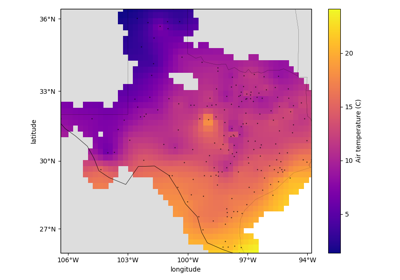Gridding with splines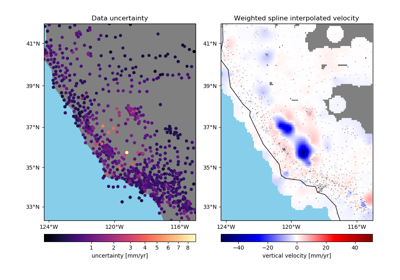Gridding with splines and weights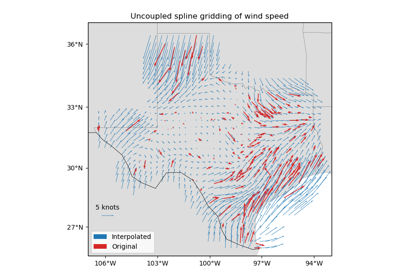Gridding 2D vectors (uncoupled)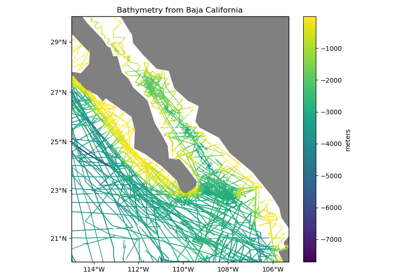Chaining Operations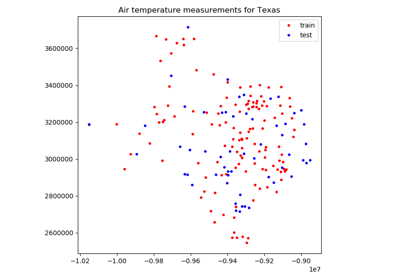Model Selection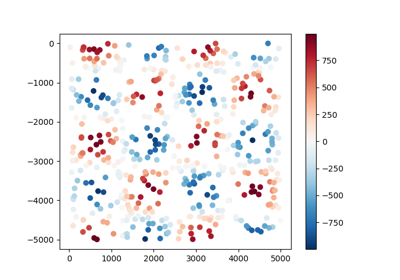Overview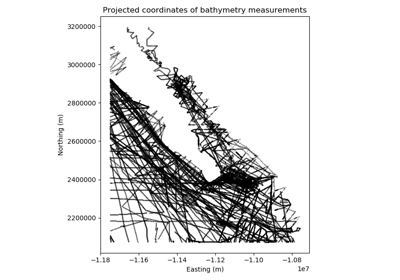Geographic Coordinates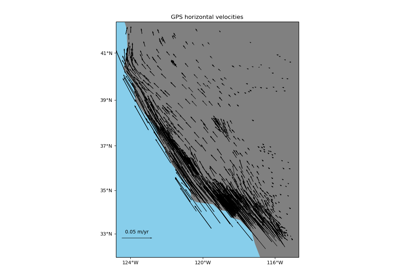Vector Data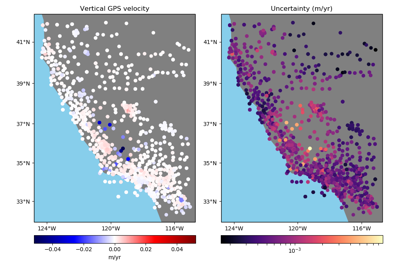Using Weights

Spline.filter(coordinates, data, weights=None)

Filter the data through the gridder and produce residuals.

Calls fit on the data, evaluates the residuals (data - predicted data), and returns the coordinates, residuals, and weights.

No very useful by itself but this interface makes gridders compatible with other processing operations and is used by verde.Chain to join them together (for example, so you can fit a spline on the residuals of a trend).

Parameters: coordinates : tuple of arrays Arrays with the coordinates of each data point. Should be in the following order: (easting, northing, vertical, …). data : array or tuple of arrays The data values of each data point. If the data has more than one component, data must be a tuple of arrays (one for each component). weights : None or array or tuple of arrays If not None, then the weights assigned to each data point. If more than one data component is provided, you must provide a weights array for each data component (if not None). coordinates, residuals, weights The coordinates and weights are same as the input. Residuals are the input data minus the predicted data.
Spline.fit(coordinates, data, weights=None)[source]

Fit the biharmonic spline to the given data.

The data region is captured and used as default for the grid and scatter methods.

All input arrays must have the same shape.

Parameters: coordinates : tuple of arrays Arrays with the coordinates of each data point. Should be in the following order: (easting, northing, vertical, …). Only easting and northing will be used, all subsequent coordinates will be ignored. data : array The data values of each data point. weights : None or array If not None, then the weights assigned to each data point. Typically, this should be 1 over the data uncertainty squared. self Returns this estimator instance for chaining operations.
Spline.get_params(deep=True)

Get parameters for this estimator.

Parameters: deep : boolean, optional If True, will return the parameters for this estimator and contained subobjects that are estimators. params : mapping of string to any Parameter names mapped to their values.
Spline.grid(region=None, shape=None, spacing=None, dims=None, data_names=None, projection=None, **kwargs)

Interpolate the data onto a regular grid.

The grid can be specified by either the number of points in each dimension (the shape) or by the grid node spacing. See verde.grid_coordinates for details. Other arguments for verde.grid_coordinates can be passed as extra keyword arguments (kwargs) to this method.

If the interpolator collected the input data region, then it will be used if region=None. Otherwise, you must specify the grid region.

Use the dims and data_names arguments to set custom names for the dimensions and the data field(s) in the output xarray.Dataset. Default names will be provided if none are given.

Parameters: region : list = [W, E, S, N] The boundaries of a given region in Cartesian or geographic coordinates. shape : tuple = (n_north, n_east) or None The number of points in the South-North and West-East directions, respectively. spacing : tuple = (s_north, s_east) or None The grid spacing in the South-North and West-East directions, respectively. dims : list or None The names of the northing and easting data dimensions, respectively, in the output grid. Defaults to ['northing', 'easting']. NOTE: This is an exception to the “easting” then “northing” pattern but is required for compatibility with xarray. data_names : list of None The name(s) of the data variables in the output grid. Defaults to ['scalars'] for scalar data, ['east_component', 'north_component'] for 2D vector data, and ['east_component', 'north_component', 'vertical_component'] for 3D vector data. projection : callable or None If not None, then should be a callable object projection(easting, northing) -> (proj_easting, proj_northing) that takes in easting and northing coordinate arrays and returns projected northing and easting coordinate arrays. This function will be used to project the generated grid coordinates before passing them into predict. For example, you can use this to generate a geographic grid from a Cartesian gridder. grid : xarray.Dataset The interpolated grid. Metadata about the interpolator is written to the attrs attribute.

verde.grid_coordinates
Generate the coordinate values for the grid.
Spline.jacobian(coordinates, force_coords, dtype='float64')[source]

Make the Jacobian matrix for the 2D biharmonic spline.

Each column of the Jacobian is the Green’s function for a single force evaluated on all observation points [Sandwell1987].

Parameters: coordinates : tuple of arrays Arrays with the coordinates of each data point. Should be in the following order: (easting, northing, vertical, …). Only easting and northing will be used, all subsequent coordinates will be ignored. force_coords : tuple of arrays Arrays with the coordinates for the forces. Should be in the same order as the coordinate arrays. dtype : str or numpy dtype The type of the Jacobian array. jacobian : 2D array The (n_data, n_forces) Jacobian matrix.
Spline.predict(coordinates)[source]

Evaluate the estimated spline on the given set of points.

Requires a fitted estimator (see fit).

Parameters: coordinates : tuple of arrays Arrays with the coordinates of each data point. Should be in the following order: (easting, northing, vertical, …). Only easting and northing will be used, all subsequent coordinates will be ignored. data : array The data values evaluated on the given points.
Spline.profile(point1, point2, size, dims=None, data_names=None, projection=None, **kwargs)

Interpolate data along a profile between two points.

Generates the profile along a straight line assuming Cartesian distances. Point coordinates are generated by verde.profile_coordinates. Other arguments for this function can be passed as extra keyword arguments (kwargs) to this method.

Use the dims and data_names arguments to set custom names for the dimensions and the data field(s) in the output pandas.DataFrame. Default names are provided.

Includes the calculated Cartesian distance from point1 for each data point in the profile.

Parameters: point1 : tuple The easting and northing coordinates, respectively, of the first point. point2 : tuple The easting and northing coordinates, respectively, of the second point. size : int The number of points to generate. dims : list or None The names of the northing and easting data dimensions, respectively, in the output dataframe. Defaults to ['northing', 'easting']. NOTE: This is an exception to the “easting” then “northing” pattern but is required for compatibility with xarray. data_names : list of None The name(s) of the data variables in the output dataframe. Defaults to ['scalars'] for scalar data, ['east_component', 'north_component'] for 2D vector data, and ['east_component', 'north_component', 'vertical_component'] for 3D vector data. projection : callable or None If not None, then should be a callable object projection(easting, northing) -> (proj_easting, proj_northing) that takes in easting and northing coordinate arrays and returns projected northing and easting coordinate arrays. This function will be used to project the generated profile coordinates before passing them into predict. For example, you can use this to generate a geographic profile from a Cartesian gridder. table : pandas.DataFrame The interpolated values along the profile.
Spline.scatter(region=None, size=300, random_state=0, dims=None, data_names=None, projection=None, **kwargs)

Interpolate values onto a random scatter of points.

Point coordinates are generated by verde.scatter_points. Other arguments for this function can be passed as extra keyword arguments (kwargs) to this method.

If the interpolator collected the input data region, then it will be used if region=None. Otherwise, you must specify the grid region.

Use the dims and data_names arguments to set custom names for the dimensions and the data field(s) in the output pandas.DataFrame. Default names are provided.

Parameters: region : list = [W, E, S, N] The boundaries of a given region in Cartesian or geographic coordinates. size : int The number of points to generate. random_state : numpy.random.RandomState or an int seed A random number generator used to define the state of the random permutations. Use a fixed seed to make sure computations are reproducible. Use None to choose a seed automatically (resulting in different numbers with each run). dims : list or None The names of the northing and easting data dimensions, respectively, in the output dataframe. Defaults to ['northing', 'easting']. NOTE: This is an exception to the “easting” then “northing” pattern but is required for compatibility with xarray. data_names : list of None The name(s) of the data variables in the output dataframe. Defaults to ['scalars'] for scalar data, ['east_component', 'north_component'] for 2D vector data, and ['east_component', 'north_component', 'vertical_component'] for 3D vector data. projection : callable or None If not None, then should be a callable object projection(easting, northing) -> (proj_easting, proj_northing) that takes in easting and northing coordinate arrays and returns projected northing and easting coordinate arrays. This function will be used to project the generated scatter coordinates before passing them into predict. For example, you can use this to generate a geographic scatter from a Cartesian gridder. table : pandas.DataFrame The interpolated values on a random set of points.
Spline.score(coordinates, data, weights=None)

Score the gridder predictions against the given data.

Calculates the R^2 coefficient of determination of between the predicted values and the given data values. A maximum score of 1 means a perfect fit. The score can be negative.

If the data has more than 1 component, the scores of each component will be averaged.

Parameters: coordinates : tuple of arrays Arrays with the coordinates of each data point. Should be in the following order: (easting, northing, vertical, …). data : array or tuple of arrays The data values of each data point. If the data has more than one component, data must be a tuple of arrays (one for each component). weights : None or array or tuple of arrays If not None, then the weights assigned to each data point. If more than one data component is provided, you must provide a weights array for each data component (if not None). score : float The R^2 score
Spline.set_params(**params)

Set the parameters of this estimator.

The method works on simple estimators as well as on nested objects (such as pipelines). The latter have parameters of the form <component>__<parameter> so that it’s possible to update each component of a nested object.

Returns: self